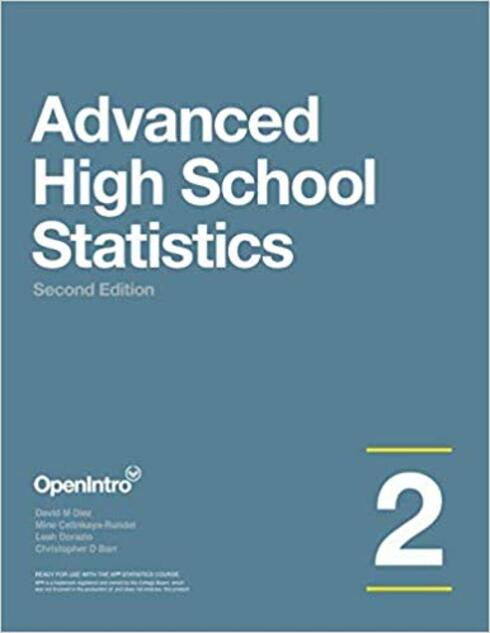# Advanced High School Statistics - 2nd Edition

(4 reviews)David Diez, OpenIntro

Mine Çetinkaya-Rundel, Duke University

Leah Dorazio, San Francisco University High School

Publisher: OpenIntro

Language: English

## Conditions of UseAttribution-ShareAlike
CC BY-SA

## Reviews

1 Data collection

• 1.1 Case study
• 1.2 Data basics
• 1.3 Overview of data collection principles
• 1.4 Observational studies and sampling strategies
• 1.5 Experiments

2 Summarizing data

• 2.1 Examining numerical data
• 2.2 Numerical summaries and box plots
• 2.3 Considering categorical data
• 2.4 Case study: malaria vaccine (special topic)

3 Probability

• 3.1 Defining probability
• 3.2 Conditional probability
• 3.3 The binomial formula
• 3.4 Simulations
• 3.5 Random variables
• 3.6 Continuous distributions

4 Distributions of random variables

• 4.1 Normal distribution
• 4.2 Sampling distribution of a sample mean
• 4.3 Geometric distribution
• 4.4 Binomial distribution
• 4.5 Sampling distribution of a sample proportion

5 Foundation for inference

• 5.1 Estimating unknown parameters
• 5.2 Confidence intervals
• 5.3 Introducing hypothesis testing
• 5.4 Does it make sense?

6 Inference for categorical data

• 6.1 Inference for a single proportion
• 6.2 Difference of two proportions
• 6.3 Testing for goodness of fit using chi-square
• 6.4 Homogeneity and independence in two-way tables

7 Inference for numerical data

• 7.1 Inference for a mean with the t-distribution
• 7.2 Inference for paired data
• 7.3 Inference for the difference of two means

8 Introduction to linear regression

• 8.1 Line fitting, residuals, and correlation
• 8.2 Fitting a line by least squares regression
• 8.3 Inference for the slope of a regression line
• 8.4 Transformations for skewed data

A Exercise solutions

B Distribution tables

C Distribution Tables

D Calculator reference, Formulas, and Inference guide

## Ancillary Material

• OpenIntro

We hope readers will take away three ideas from this book in addition to forming a foundationof statistical thinking and methods.

• (1) Statistics is an applied field with a wide range of practical applications.
• (2) You don't have to be a math guru to learn from real, interesting data.
• (3) Data are messy, and statistical tools are imperfect. But, when you understand the strengths and weaknesses of these tools, you can use them to learn about the real world.

Textbook overviewThe chapters of this book are as follows:

• 1. Data collection. Data structures, variables, and basic data collection techniques.
• 2. Summarizing data. Data summaries and graphics.
• 3. Probability. The basic principles of probability.
• 4. Distributions of random variables. Introduction to key distributions, and how the normal model applies to the sample mean and sample proportion.
• 5. Foundation for inference. General ideas for statistical inference in the context of estimating the population proportion.
• 6. Inference for categorical data. Inference for proportions using the normal and chisquare distributions.
• 7. Inference for numerical data. Inference for one or two sample means using the t distribution, and comparisons of many means using ANOVA.
• 8. Introduction to linear regression. An introduction to regression with two variables.

Instructions are also provided in several sections for using Casio and TI calculators.

### Authors

David Diez is a Data Scientist at OpenIntro.

Christopher Barr is an Investment Analyst at Varadero Capital.

Dr. Mine Çetinkaya-Rundel is the Director of Undergraduate Studies and an Associate Professor of the Practice in the Department of Statistical Science at Duke University. She received her Ph.D. in Statistics from the University of California, Los Angeles, and a B.S. in Actuarial Science from New York University’s Stern School of Business. Her work focuses on innovation in statistics pedagogy, with an emphasis on student-centered learning, computation, reproducible research, and open-source education.

Leah Dorazio, Statistics and Computer Science Teacher, San Francisco University High School